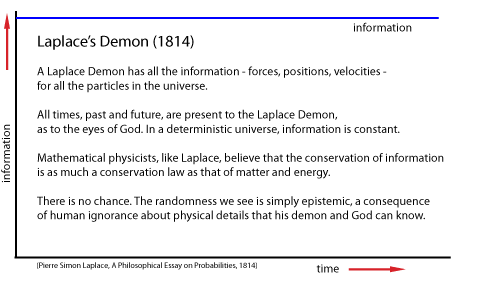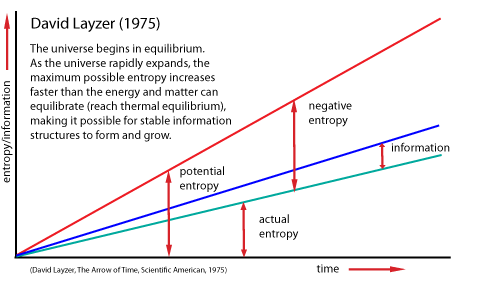Home > Solutions > Scientists > Zermelo Ernst Zermelo (1871-1953) Ernst Zermelo was a brilliant mathematician who developed what is now known as ZFC (Zermelo-Fraenkel-Choice) axiomatic set theory, a major contribution to the foundations of mathematics. In the 1890's, after finishing his doctorate at the University of Berlin, he took a position as assistant to Max Planck, with whom he developed a criticism of Ludwig Boltzmann's H-Theorem which is known as the Zermelo recurrence objection. The H-Theorem was Boltzmann's attempt to derive the increasing entropy required by the second law of thermodynamics from basic classical dynamics. It was the second "paradox" or "objection" attack on Boltzmann. The first was Josef Loschmidt's claim that entropy would be reduced if time were reversed. This is the problem of microscopic reversibility. Boltzmann reformulated his H-Theorem on purely statistical grounds in response to the Loschmidt objection, but twenty years later he was faced with an objection even to his statistical arguments. Ernst Zermelo was an extraordinary mathematician. He was (in 1908) the founder of axiomatic set theory, which with the addition of the axiom of choice (also by Zermelo, in 1904) is the most common foundation of mathematics. The axiom of choice says that given any collection of sets, one can find a way to unambiguously select one object from each set, even if the number of sets is infinite. Before this amazing work, Zermelo was a young associate of Max Planck in Berlin, where many German physicists (notably led by the energeticist Wilhelm Ostwald and physicist-philosopher Ernst Mach) were opposed to the work of Boltzmann to establish the existence of atoms. Planck was an expert in the three-body problem, which, unlike the problem of two particles, has no exact analytic solution. Planck had been able to establish limits or bounds on the possible configurations of the three bodies from conservation laws. He and Zermelo applied some of this thinking to the n particles in a gas. They argued that given a long enough time, the particles would return to a distribution in "phase space" (a 6n dimensional space of possible velocities and positions) that would be indistinguishable from the original distribution. Thus, they argued, Boltzmann's formula for the entropy would at some future time go back down, vitiating Boltzmann's claim that his measure of entropy always increases - as the second law of thermodynamics requires. Boltzmann replied that his argument was statistical. He only claimed that entropy increase was overwhelmingly more probable than Zermelo's predicted decrease. Boltzmann calculated the probability of a decrease of a very small gas of only a few hundred particles and found the time needed to realize such a decrease was many orders of magnitude larger than the presumed age of the universe. The idea that a macroscopic system can return to exactly the same physical conditions is closely related to the idea that an agent may face "exactly the same circumstances in making a decision. Determinists maintain that given the "fixed past" and the "laws of nature" that the agent would have to make exactly the same decision again. The Extreme Improbability of Perfect Recurrence In a classical universe, such as that of Laplace, where information is constant, Zermelo's recurrence is mathematically possible. The universe can return to the exact circumstance of any earlier instant of time, because it contains the same amount of matter, energy, and information.But, in the real universe, information (and the material content of the universe) expands from a minimum at the origin, to ever larger amounts of information - as well as increased entropy, of course. Consequently, it is statistically and realistically improbable (if not impossible) for the universe to return to exactly the same circumstance of any earlier time.For Teachers To hide this material, click on the Normal link. Noesis PhilPapers Stanford Encyclopedia of Philosophy Wikipedia For Scholars To hide this material, click on the Teacher or Normal link. Normal | Teacher | Scholar